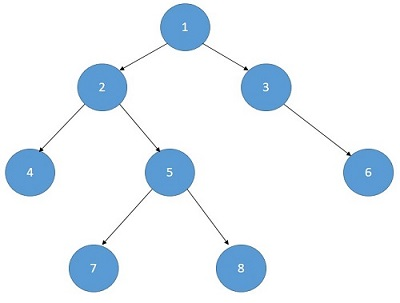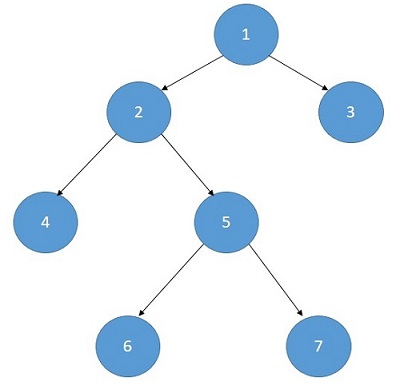Q:

# Largest Independent Set Problem

Given a tree, you have to find out the largest independent set. Independent elements are those that don’t have any common edges. Print the length of the largest independent set.

```T Test case
T no. of input string will be given to you.

E.g.
2Output:
Print the length of the largest Independent set.
```

Example

```T=2

Input:Output:
5 ( 1,4,7,8,6)

Input:Output:
4 (3,2,6,7)
```

Explanation with example:

To find out the length of the independent set we have to consider every node in our consideration.

• A node with its grandchildren are in the set
• The child nodes are in the set.

Independent set of node(x) = max⁡ (1+independent set of its grandchildren,independent set of its children)

Example:

```In that case,
Independent set of the node(1) =
max ⁡( independent set of node(4), node(5) and node(6)+1 ,
independent set of the node(2)and node(3)```

Recursive algorithm:

```Function(Node):
if(node==NULL)
return 0
excluding_the_node=Function(node->left)+Function(node->right)
including_the_node=1
if(node->left)
including_the_node+=Function(node->left->left)+Function(node->left->right)
if(node->right)
including_the_node+=Function(node->right->left)+Function(node->right->right)
return max(including_the_node,excluding_the_node)
```

DP conversion:

```Function(Node):
if(node==NULL)
return 0
if(vis[node])
return vis[node]
excluding_the_node=Function(node->left)+Function(node->right)
including_the_node=1
if(node->left)
including_the_node+=Function(node->left->left)+Function(node->left->right)
if(node->right)
including_the_node+=Function(node->right->left)+Function(node->right->right)
return vis[node]=max(including_the_node,excluding_the_node)
```

C++ Implementation:

``````#include <bits/stdc++.h>
using namespace std;

struct tree {
int val, value;
tree* left;
tree* right;
};

struct tree* make_node(int x)
{
tree* t = new tree;
t->val = x;
t->value = 0;
t->left = t->right = NULL;
return t;
}

int set_count(tree* t)
{
if (t == NULL) {
return 0;
}
if (t->value) {
return t->value;
}
int excluding = set_count(t->left) + set_count(t->right);
int including = 1;
if (t->left)
including += set_count(t->left->left) + set_count(t->left->right);
if (t->right)
including += set_count(t->right->left) + set_count(t->right->right);
return max(excluding, including);
}

int main()
{

tree* t = NULL;
t = make_node(10);
t->left = make_node(20);
t->right = make_node(30);
t->left->left = make_node(40);
t->left->right = make_node(50);
t->right->right = make_node(60);
t->right->left = make_node(90);
t->left->right->left = make_node(70);
t->left->right->right = make_node(80);

cout << "Max set count : " << set_count(t) << endl;

return 0;
}``````

Output

`Max set count : 6`